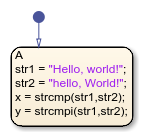# strcmpi

Compare strings (case insensitive)

## Syntax

``tf = strcmpi(s1,s2)``

## Description

example

````tf = strcmpi(s1,s2)` compares `s1` and `s2`, ignoring any differences in letter case. The function returns `1` (`true`) if the two are identical and `0` (`false`) otherwise. Text is considered identical if the size and content of each are the same, aside from case. The return result `tf` is of data type `logical`.```

Note

The operator `strcmpi` is supported only in Stateflow® charts that use MATLAB® as the action language.

## Examples

expand all

Return a value of `0` (strings are equal).

```h1 = "Hello"; h2 = "Hello"; h3 = "hello"; x = strcmpi(h1,h2) y = strcmpi(h1,h3)```## Version History

Introduced in R2021b